CIM Created Edited

# [CIM/Rebar] Rebar Array Group Creation Rule according to Transverse Rebar Draw Point.

## Question

When transverse rebars are arrayed, rebars are arrayed differently than intended. Is it possible to arbitrarily modify it?

You can change the arrangement of rebars by drawing rebars by changing the position of the Draw Point.

Info

When you understand this and create a rebar, you will be able to proceed with more efficient rebar modeling.
There are various Draw Types within the function, but basically all Transverse Rebars have Draw Points.
Here, the Draw point is the reference point used to draw one Transverse Rebar. [One]
Array works by drawing new reinforcing bars one by one based on the position of the draw point. 
Therefore, each of the arrayed reinforcing bars has shape information, and the information that determines the rebar shape is as follows.

Check the draw point of the pro-created reinforcing bar
You can check the draw point of the drawn rebar through
Array Rebar > Type(Sweep Array) > Select rebar.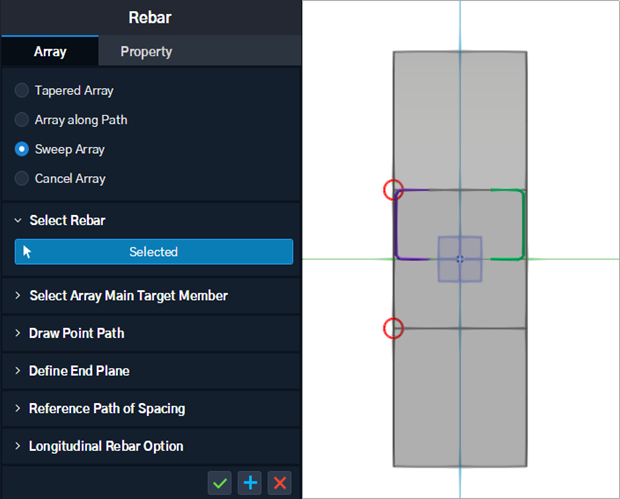As shown in the figure, the shape of the reinforcing bar (half of the edge) and the draw point (both ends of the edge) may be different.
Rebar Array Action Sequence.
1. As shown in the figure, the shape of the reinforcing bar (half of the edge) and the draw point
(both ends of the edge) may be different.
2. In each section, the same draw point as the target rebar is traced.
3. Draw a rebar connecting each point.
(Even in the case of a side section, a variable rebar group is created by tracing the draw point automatically.)

Information that determines the rebar shape
The user creates the final reinforcing bar shape through property settings such as offset based on the draw point.

In summary, there are two pieces of information that determine the shape of the reinforcing bar.

Draw Point : As the section changes, the position changes according to a specific rule.
Property : Fixed information to be reflected in the shape regardless of cross-sectional changes.

Therefore, for reinforcement work in CIM, it is not necessary to import the reinforcement diagram and draw the reinforcement only with the Select line type.

Only when the information of the rebar to move in 3D is properly combined and drawn as needed, the user can obtain the desired modeling result.

Then, let's see how the draw point moves according to the change of the section in the side section object. The rules are divided into two depending on whether a Point exists on an edge or not.

Case 1.
When the Point exists on the Edge: Arranged along the Ratio on the Edge.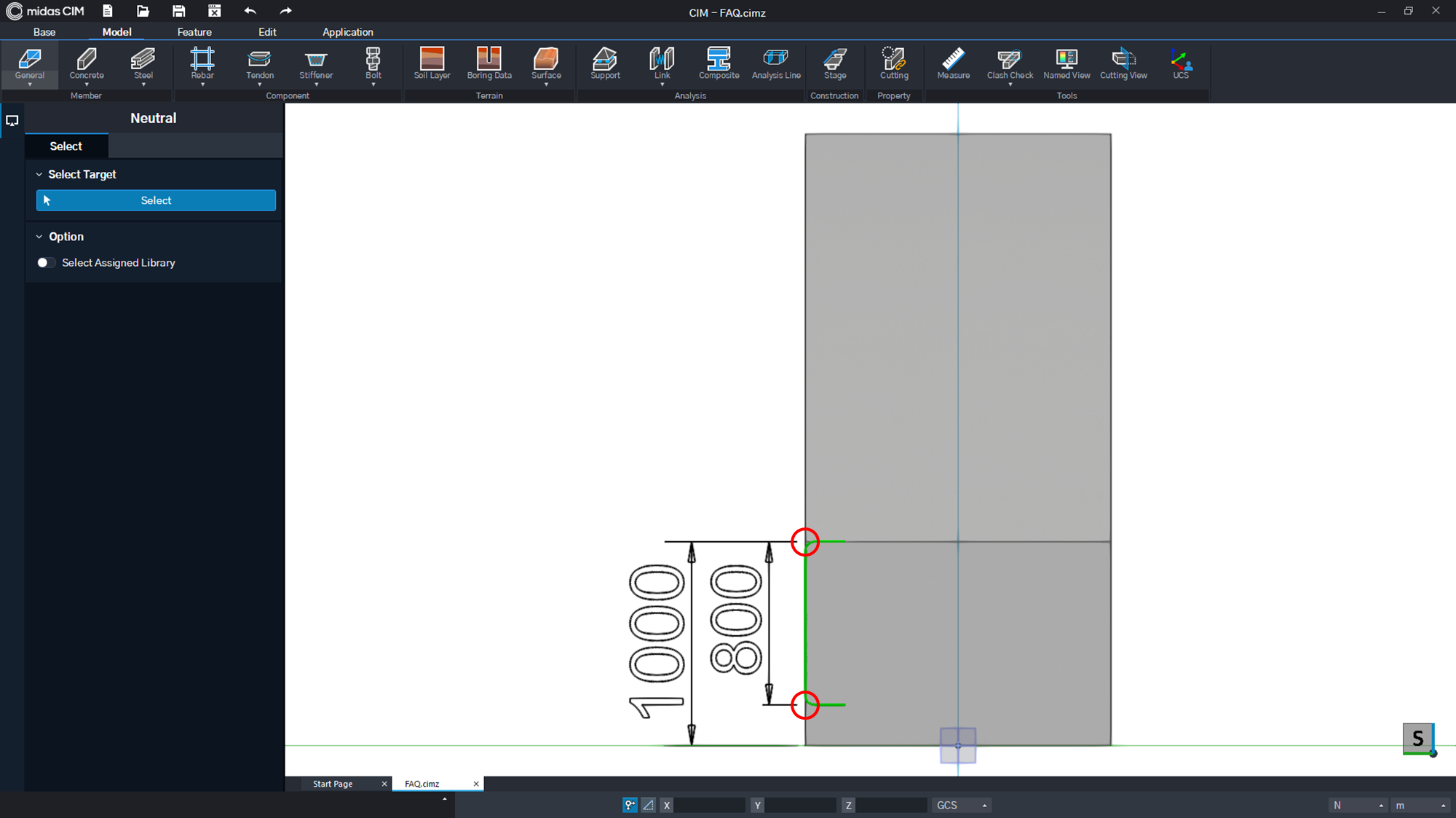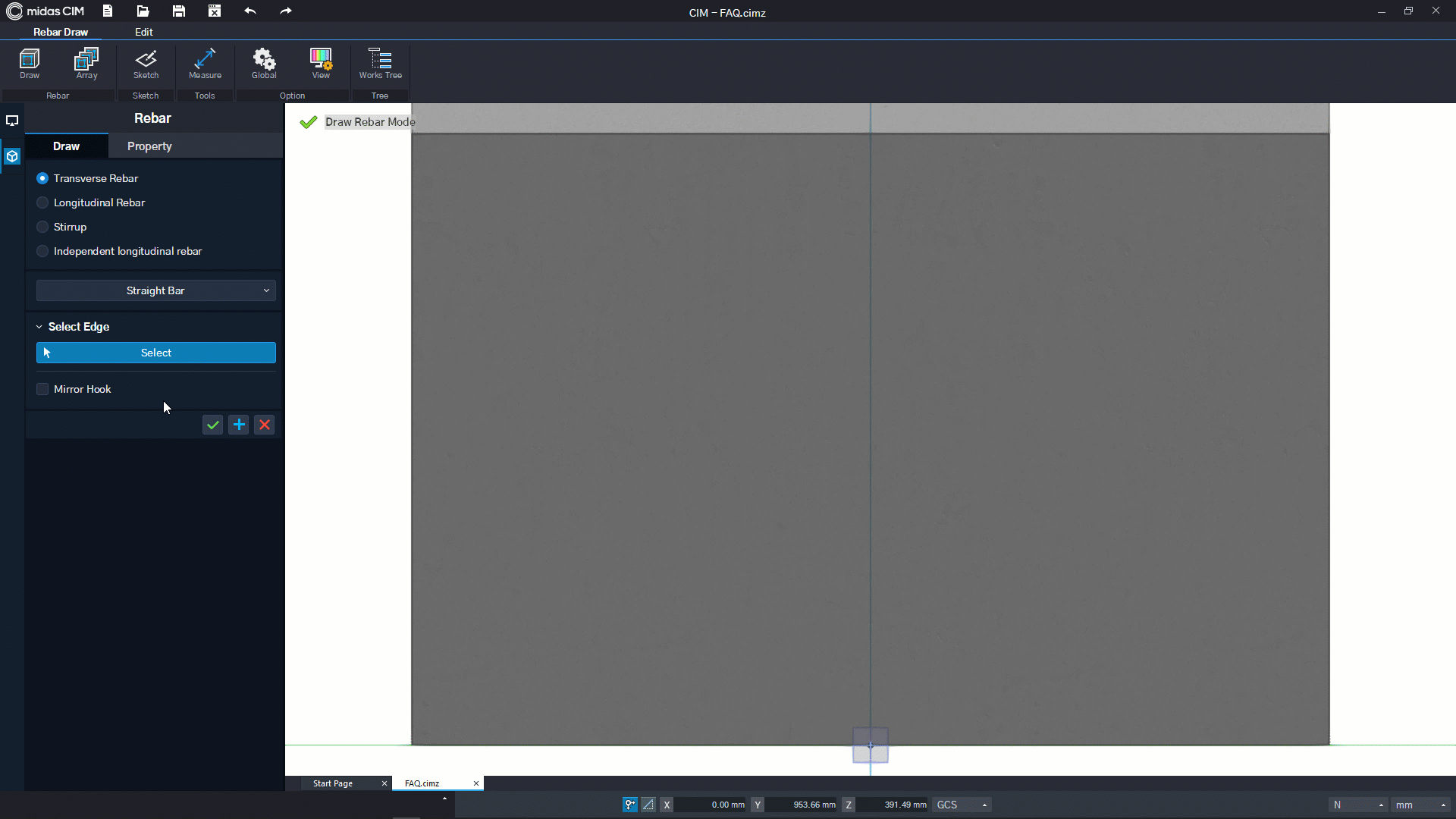Figure. 1 Draw point Ratio (0.8) on 1 edge Figure. 2 Result arrayed according to Ratio(0.8)

Figure. 1 The reinforcing bar of 1 is a reinforcing bar using the two points on the edge as draw points. Each first point is a reinforcing bar with the upper edge (Ratio=0) and the second point 4/5 points (Ratio=0.8) of the edge as information.

In this case, if you proceed with Tapered Array, it finds the same edge in all sections and draws reinforcing bars connecting Ratio 0~0.8 points.

Case 2.
When a Point exists outside the Edge: Arranged along the Distance of the adjacent Point.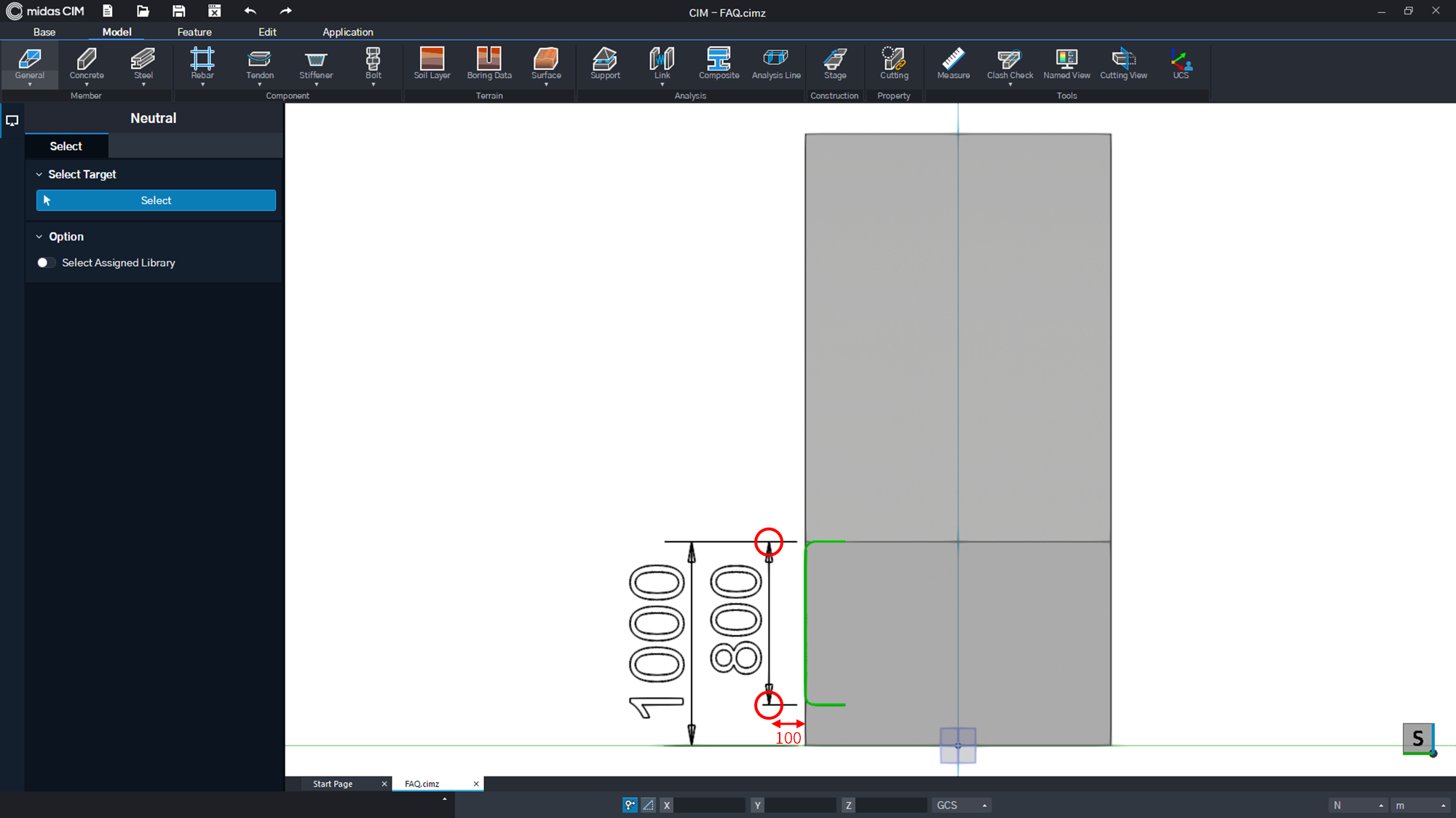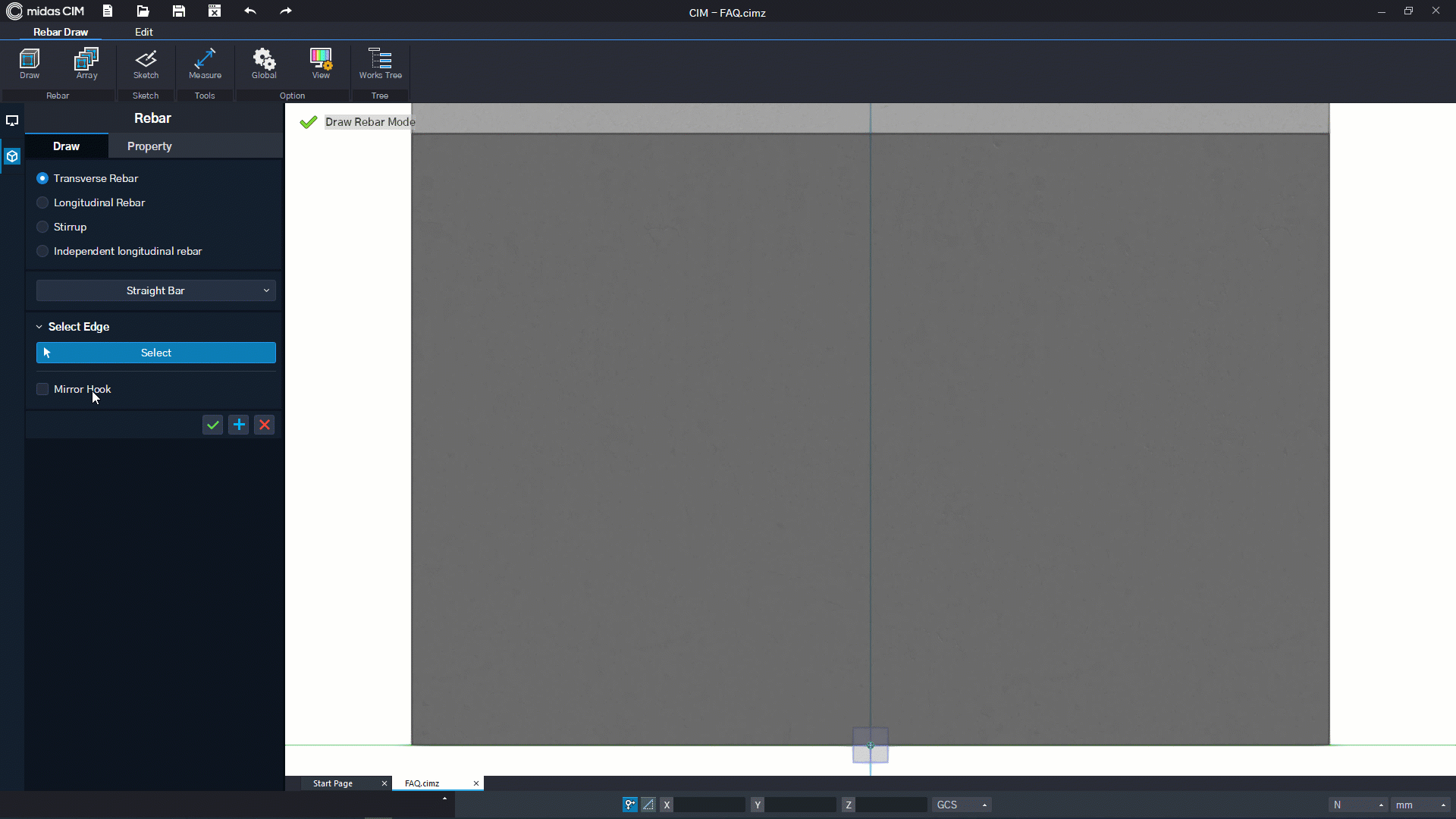Figure. 3 Draw point distance outside the edge Figure. 4 Result Arrayed along Distance

Figure. The reinforcing bar in 3 is a reinforcing bar using two points outside the edge as draw points. Each Point exists outside the object as shown.

In this case, the draw point has information in relative coordinates (x,y distance) from the nearest point on the section.

In this example, First Point is a relative distance from the upper left point (x=-0.1m, y=0.0m), and the Second Point is a relative distance from the lower left point by (x=-0.1m, y=0.2m).

Therefore, in Tapered Array, the same point is found in all cross sections and a reinforcing bar is drawn from the point to the relative coordinate point.

In this way, if you fully understand the information rules that determine the shape of the Transverse Rebar and then work, you can model the reinforcing bars of various cases as desired by the user.

0